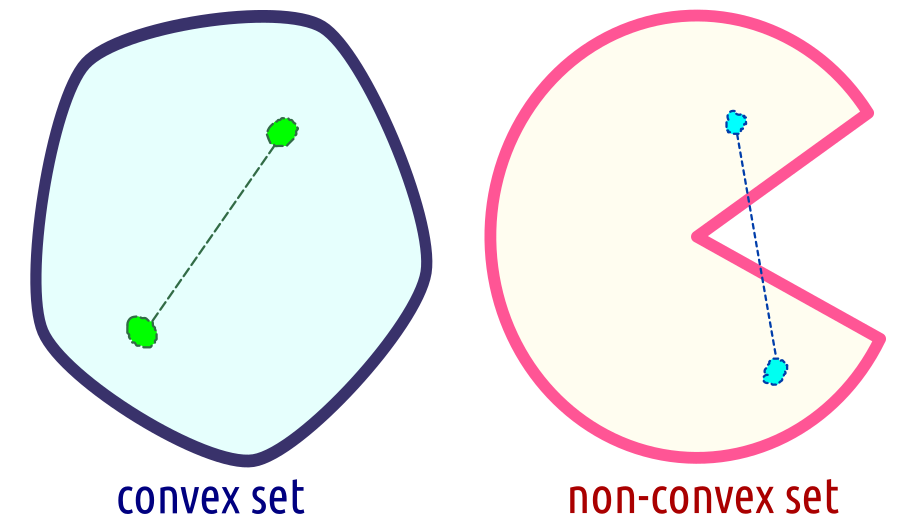## 8.2 A Note on Convex Optimisation (*)

### 8.2.1 Introduction

Are there cases where we are sure that a local minimum is the global minimum?

Yes. For example when we minimise convex objective functions.

Here is just a very brief overview of the topic for the interested, see also (Boyd & Vandenberghe 2004) for more.

For example, the linear regression or the logistic regression have convex objectives – they are very well-behaving.

Note that this doesn’t mean that we know an analytic solution.

### 8.2.2 Convex Combinations (*)

A convex combination of a set of points $$x_1,\dots,x_n\in D$$ is a linear combination $\theta_1 x_1 + \theta_2 x_2 + \dots + \theta_n x_n$ for some $$\theta_1,\theta_2,\dots,\theta_n$$ that fulfils $$\theta_1,\theta_2,\dots,\theta_n\ge 0$$ and $$\theta_1+\theta_2+\dots+\theta_n=1$$.

Think of this as a weighted arithmetic mean of these points.The set of all convex combinations of two points $$x_1,x_2\in D$$: $\{\theta x_{1}+(1-\theta)x_{2}: \theta\in [0,1]\}$ is just the line segment between these two points.The set of all convex combinations of 3 points yields a triangle (unless $$D$$ is one-dimensional).More generally, we get the convex hull of a set of points – the smallest set $$C$$ enclosing all the points that is convex, i.e., a line segment between any two points in $$C$$ is fully included in $$C$$.

In two dimensions, think of a rubber band stretched around all the points like a fence.### 8.2.3 Convex Functions (*)

We call a function $$f:D\to\mathbb{R}$$ convex, whenever: $(\forall x_{1},x_{2}\in D) (\forall \theta\in [0,1])\quad f(\theta x_{1}+(1-\theta)x_{2})\leq \theta f(x_{1})+(1-\theta)f(x_{2})$(function value at any convex combination of two points is not greater than that combination of the function values at these two points)Convex: $$f(\theta x_{1}+(1-\theta)x_{2})\leq \theta f(x_{1})+(1-\theta)f(x_{2})$$

Concave: $$f(\theta x_{1}+(1-\theta)x_{2})\geq \theta f(x_{1})+(1-\theta)f(x_{2})$$

Theorem

For any convex function $$f$$, if $$f$$ has a local minimum at $$x$$ then $$x$$ is also its global minimum.

Optimising convex functions is relatively easy, especially if they are differentiable.

Methods such as gradient descent or BFGS should work very well (unless there are large regions where a function is constant…).

(**) There is a special class of constrained optimisation problems called linear and quadratic programming that involves convex functions, see (Nocedal & Wright 2006, Fletcher 2008).

(***) See also the Karush–Kuhn–Tucker (KKT) conditions for a more general problem of minimisation with constraints.

### 8.2.4 Examples

• $$x^2, |x|, e^x$$ are all convex.
• $$|x|^p$$ is convex for all $$p\ge 1$$.
• if $$f$$ is convex, then $$-f$$ is concave.
• if $$f_1$$ and $$f_2$$ are convex, then $$w_1 f_1 + w_2 f_2$$ are convex for any $$w_1,w_2\ge 0$$.
• if $$f_1$$ and $$f_2$$ are convex, then $$\max\{f_1, f_2\}$$ is convex.
• if $$f$$ and $$g$$ are convex and $$g$$ is non-decreasing, then $$g(f(x))$$ is convex.
• Sum of squared residuals in linear regression is a convex function of the underlying parameters.
• Cross-entropy in logistic regression is a convex function of the underlying parameters.